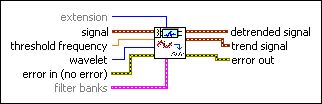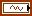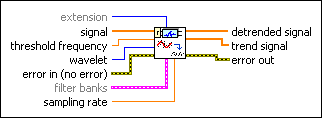# WA Detrend VI

LabVIEW 2014 Advanced Signal Processing Toolkit Help

Edition Date: June 2014

Part Number: 372656C-01

»View Product InfoDownload Help (Windows Only)

Owning Palette: Feature Extraction VIs

Removes the trend from 1D signals by setting the approximation coefficients to zeros. Wire data to the signal input to determine the polymorphic instance to use or manually select the instance.Note  Use this VI for offline wavelet analysis. To remove the trend from streaming signals, use the WA Online Detrend VI.

Use the pull-down menu to select an instance of this VI.

 Select an instance WA Detrend (Waveform)WA Detrend (Array)

## WA Detrend (Waveform)extension specifies the method to use to pad data at the borders of the input signal. The extension length is equal to the length of the wavelet filters. When you select the extension method, make the transition between the input signal and the padded data as smooth as possible because a smooth transition generates fewer large detail coefficients and enhances the efficiency of the signal representation.

 0 Zero padding—Uses zeros to pad the input data. Watch for abrupt transitions between the padded zeros and the input data, which causes large artifacts near the transition. 1 Symmetric (default)—Uses replications of the input data to pad the data, except that this VI left-flips the block at the input and right-flips the block at the end. 2 Periodic—Adds a replication of the input data block before and another replication after the input data block to pad the data.signal specifies the input signal.threshold frequency specifies the upper frequency limit, in hertz, of the trend that this VI removes from the signal. The threshold frequency determines the wavelet transform level. The wavelet transform level specifies the number of levels in the discrete wavelet analysis. The wavelet transform level is floor(log2[sampling rate/(2*threshold frequency)]). The floor function rounds a value to the nearest integer towards negative infinity. The default is –1, which means this VI sets the threshold frequency automatically.wavelet specifies the wavelet type to use for the discrete wavelet analysis. The default is db02. The options include two types: orthogonal (Haar, Daubechies (dbxx), Coiflets (coifx), Symmlets (symx)) and biorthogonal (Biorthogonal (biorx_x), including FBI (bior4_4 (FBI))), where x indicates the order of the wavelet. The higher the order, the smoother the wavelet. The orthogonal wavelets are not redundant and are suitable for signal or image denoising and compression. The biorthogonal wavelets usually have the linear phase property and are suitable for signal or image feature extraction. If you want to use other types of wavelets, do not wire this input. Instead, use the Wavelet Design Express VI to design the wavelet you want, bundle the resulting analysis and synthesis filters, and then wire them to the filter banks input.error in describes error conditions that occur before this node runs. This input provides standard error in functionality.filter banks specifies the analysis filter banks and the synthesis filter banks for the wavelet you specify. If you specify a value for filter banks, this VI ignores the settings in the wavelet input. You can use the Wavelet Design Express VI to design the analysis filters and the corresponding synthesis filters.analysis filters specifies the coefficients of the lowpass analysis filters and the highpass analysis filters for the wavelet you specify.lowpass specifies the coefficients of the lowpass analysis filter, which this VI uses to compute the approximation coefficients.highpass specifies the coefficients of the highpass analysis filter, which this VI uses to compute the detail coefficients.synthesis filters specifies the coefficients of the lowpass synthesis filters and the highpass synthesis filters for the wavelet you specify.lowpass specifies the coefficients of the lowpass synthesis filter, which this VI uses to filter the interpolated approximation coefficients in the wavelet reconstruction.highpass specifies the coefficients of the highpass synthesis filter, which this VI uses to filter the interpolated detail coefficients in the wavelet reconstruction.detrended signal returns the signal without the trend.trend signal returns the residual trend of the signal.error out contains error information. This output provides standard error out functionality.

## WA Detrend (Array)extension specifies the method to use to pad data at the borders of the input signal. The extension length is equal to the length of the wavelet filters. When you select the extension method, make the transition between the input signal and the padded data as smooth as possible because a smooth transition generates fewer large detail coefficients and enhances the efficiency of the signal representation.

 0 Zero padding—Uses zeros to pad the input data. Watch for abrupt transitions between the padded zeros and the input data, which causes large artifacts near the transition. 1 Symmetric (default)—Uses replications of the input data to pad the data, except that this VI left-flips the block at the input and right-flips the block at the end. 2 Periodic—Adds a replication of the input data block before and another replication after the input data block to pad the data.signal specifies the input signal.threshold frequency specifies the upper frequency limit, in hertz, of the trend that this VI removes from the signal. The threshold frequency determines the wavelet transform level. The wavelet transform level specifies the number of levels in the discrete wavelet analysis. The wavelet transform level is floor(log2[sampling rate/(2*threshold frequency)]). The floor function rounds a value to the nearest integer towards negative infinity. The default is –1, which means this VI sets the threshold frequency automatically.wavelet specifies the wavelet type to use for the discrete wavelet analysis. The default is db02. The options include two types: orthogonal (Haar, Daubechies (dbxx), Coiflets (coifx), Symmlets (symx)) and biorthogonal (Biorthogonal (biorx_x), including FBI (bior4_4 (FBI))), where x indicates the order of the wavelet. The higher the order, the smoother the wavelet. The orthogonal wavelets are not redundant and are suitable for signal or image denoising and compression. The biorthogonal wavelets usually have the linear phase property and are suitable for signal or image feature extraction. If you want to use other types of wavelets, do not wire this input. Instead, use the Wavelet Design Express VI to design the wavelet you want, bundle the resulting analysis and synthesis filters, and then wire them to the filter banks input.error in describes error conditions that occur before this node runs. This input provides standard error in functionality.filter banks specifies the analysis filter banks and the synthesis filter banks for the wavelet you specify. If you specify a value for filter banks, this VI ignores the settings in the wavelet input. You can use the Wavelet Design Express VI to design the analysis filters and the corresponding synthesis filters.analysis filters specifies the coefficients of the lowpass analysis filters and the highpass analysis filters for the wavelet you specify.lowpass specifies the coefficients of the lowpass analysis filter, which this VI uses to compute the approximation coefficients.highpass specifies the coefficients of the highpass analysis filter, which this VI uses to compute the detail coefficients.synthesis filters specifies the coefficients of the lowpass synthesis filters and the highpass synthesis filters for the wavelet you specify.lowpass specifies the coefficients of the lowpass synthesis filter, which this VI uses to filter the interpolated approximation coefficients in the wavelet reconstruction.highpass specifies the coefficients of the highpass synthesis filter, which this VI uses to filter the interpolated detail coefficients in the wavelet reconstruction.sampling rate specifies the sampling rate of signal in hertz. sampling rate must be greater than 0, or this VI sets sampling rate to 1 automatically. The default is 1.detrended signal returns the signal without the trend.trend signal returns the residual trend of the signal.error out contains error information. This output provides standard error out functionality.

## WA Detrend Details

The trend of the input signal is the slow-varying part of the signal that mainly contributes to the approximation coefficients. This VI applies the following steps to implement the detrend function.

1. Applies the discrete wavelet transform (DWT) to the input signal.
2. Sets the approximation coefficients to 0.
3. Reconstructs the signal based on all the detail coefficients.

## Example

Refer to the Detrend and Trend Estimation VI in the labview\examples\Wavelet Analysis\WAGettingStarted directory for an example of using the WA Detrend VI.